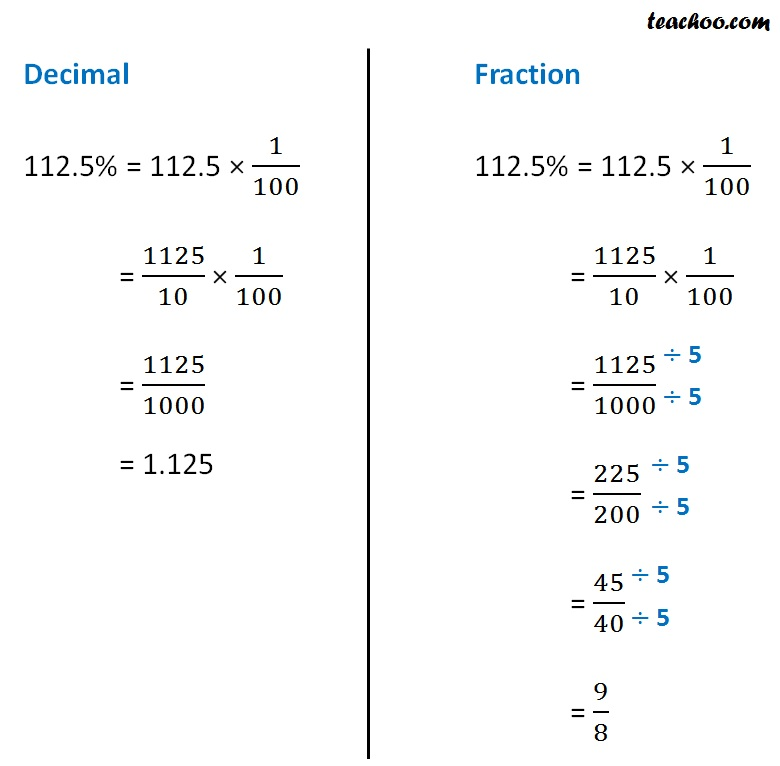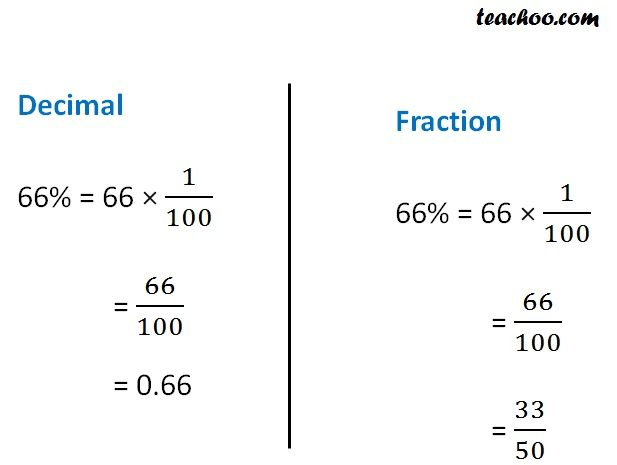Percentage to Fractions and Decimals

Chapter 7 Class 7 Comparing Quantities
Concept wise

Suppose I have percentage

50%

How do I convert into decimals and fractions?

We know that

% = 1/100

So, we write 1/100 in place of % & calculate

50% = 50 × 1/100

= 50/100

= 0.50

And to convert into fractions,

50% = 0.50

= 50/100

Simplifying

1/2

#### Convert 112.5% into decimals & fractions#### Convert 66% into decimals & fractionsLearn in your speed, with individual attention - Teachoo Maths 1-on-1 Class